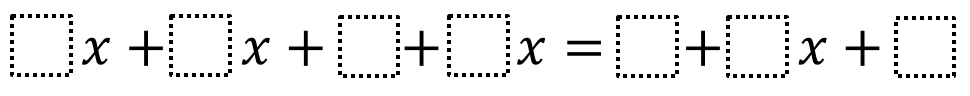Home > Grade 6 > Expressions & Equations > Equivalent Expressions 1

# Equivalent Expressions 1

Directions: Using the digits 1 to 9 at most one time each, fill in the boxes to create two expressions that are equivalent to one another.### Hint

What values will make the constants equivalent? What values will make the variable terms equivalent?

### Answer

Here are some possibilities:
1x + 2x + 9 + 3x = 4 + 6x + 5;
1x + 2x + 8 + 4x = 3 + 7x + 5

Source: Will Case

## Subtracting Multi-Decimals

Directions: Use the digits 1 to 9, at most one time each, to fill in …

### One comment

1.answer 3x+2x+8+4x=1+9x+7